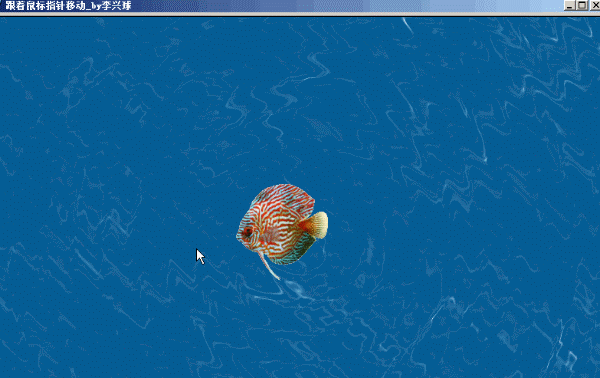### python海龟画图模块制作的水波纹模拟和跟着鼠标指针前进的左右造型的鱼

```'''水波纹模拟和跟着鼠标指针前进的左右造型的鱼.py '''
import math
from turtle import Turtle,Screen     # 导入Turtle类和Screen命令
from random import randint

def animate_fish():
"""鱼切换造型"""
if fish.costume_index < fish.costume_amounts:
image = fish.images[fish.costume_index]
fish.shape(image)
fish.costume_index += 1
fish.costume_index = fish.costume_index % fish.costume_amounts
screen.ontimer(animate_fish,100)

def animate_screen():
"""背景切换"""
global bg_index
screen.bgpic(waveimages[bg_index])
bg_index = bg_index + 1
bg_index = bg_index % bg_amounts
screen.ontimer(animate_screen,100)

if __name__ == "__main__":

bg_index = 0
width,height = 800,600

fish_right_images = ["images/fish_right0.gif","images/fish_right1.gif"]
fish_left_images = ["images/fish_left0.gif","images/fish_left1.gif"]

fish_right_images = ["fishs/" + "0" * (4-len(str(i))) + str(i) +".gif" for i in range(1,9)]
fish_left_images = ["fishs/" + "0" * (4-len(str(i))) + str(i) +"-.gif" for i in range(1,9)]

fish_images = [fish_left_images,fish_right_images]

#waveimages = ["waves/" + (4-len(str(i))) * "0" + str(i) + ".png" for i in range(1,21)]
waveimages = [f"waves/{i:04d}.png" for i in range(1,21)]
bg_amounts = len(waveimages)

screen = Screen()
screen.title('跟着鼠标指针移动_by李兴球')     # 写上窗口标题
screen.setup(width,height)           # 设定窗口大小
screen.delay(0)
[screen.addshape(image) for image in fish_right_images]
[screen.addshape(image) for image in fish_left_images]

ball = Turtle(shape='circle',visible=False)
ball.penup()
ball.speed(0)
ball.color('cyan')

fish = Turtle(shape = "turtle")
fish.penup()
fish.speed(0)
fish.left_right = 0                       # 用左边的图还是右边的图,0左,1右
fish.images = fish_right_images           # 初始用右边的一系列图
fish.costume_index = 0                    # 自定义一个属性，用于造型切换索引
fish.costume_amounts = len(fish_right_images) # 一个方向的造型数量，两个方向是一样的数量
animate_fish()
animate_screen()
screen.cv.bind("<Motion>",follow_mouse)   # 画布绑定鼠标移动事件
screen.mainloop()

```